Courses

# Huygens Principle Interference of Light Waves and Young's Experiment Class 12 Notes | EduRev

## Class 12 : Huygens Principle Interference of Light Waves and Young's Experiment Class 12 Notes | EduRev

The document Huygens Principle Interference of Light Waves and Young's Experiment Class 12 Notes | EduRev is a part of the Class 12 Course Physics For JEE.
All you need of Class 12 at this link: Class 12

Wave Optics

Wave optics describes the connection between waves and rays of light. According to the wave theory of light, the light is a form of energy which travels through a medium in the form of transverse wave motion. The speed of light in a medium depends upon the nature of the medium.

Newton’s Corpuscular Theory

• Light consists of very small invisible elastic particles which travel in vacuum with a speed of 3 x 108 m/s.
• The theory could explain reflection and refraction.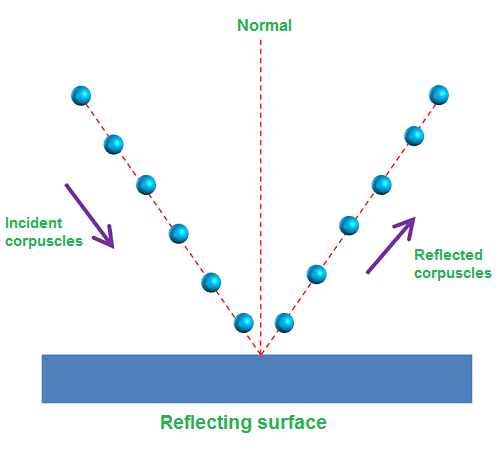Newton's Corpuscular Theory

• The size of corpuscular of different colours of light are different.
• It could not explain interference, diffraction, polarization. photoelectric effect and Compton effect. The theory failed as it could not explain why light travels faster in a rarer medium than in a denser medium.

Wavefront

A wavefront is defined as the continuous locus of all the particles of a medium, which are vibrating in the same phase.

These are three types:

• Spherical wavefront
• Cylindrical wavefront
• Plane wavefront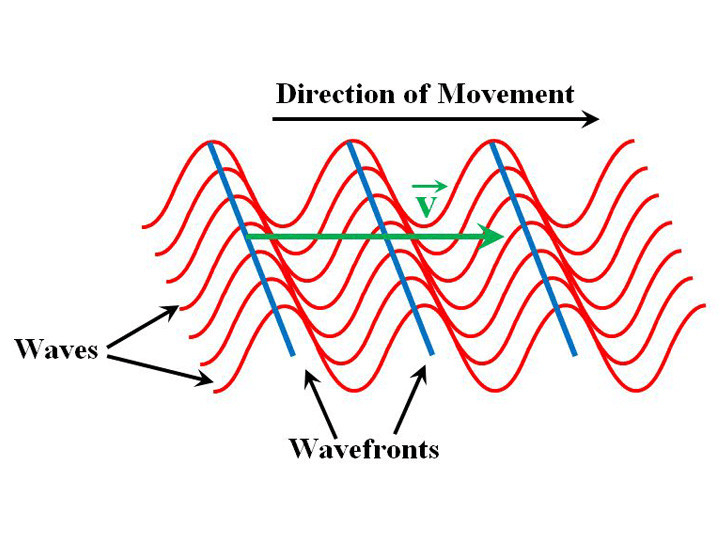S = source of light.
AB = wavefront and SP SQ and SR are rays cf light

Huygen’s Wave Theory

• Light travel in a medium in the form of wavefront.
• A wavefront is the locus of all the particles vibrating in same phase.
• All particles on a wavefront behaves as a secondary source of light, which emits secondary wavelets.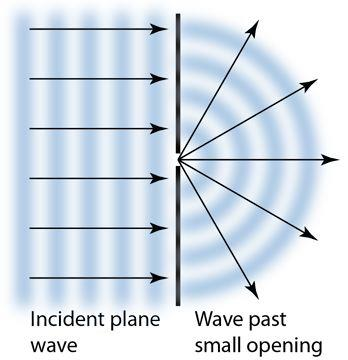Huygen's Principle

• The envelope of secondary wavelets represents the new position of a wavefront.
• When source of light is a point source,the wavefront is spherical.
• Amplitude (A) is inversely proportional to distance (x) i.g., A ∝ 1 / x .
∴ Intensity (I) ∝ (Amplitude)2
• When Source of light is linear, the wavefront is cylindrical.
• Amplitude (A) ∝ 1 / √x
∴ Intensity ∝ (Amplitude)2 ∝ 1 / x

Huygen’s Principle

• Every point on given wavefront (called primary wavefront) acts as a fresh source of new disturbance called secondary wavelets.
• The secondary wavelets travels in all the directions with the speed of light in the medium.
• A surface touching these secondary wavelets tangentially in the forward direction at any instant gives the new (secondary) wave front of that instant.

Maxwell’s Electromagnetic Wave Theory

• Light waves are electromagnetic waves which do not require a material medium for their propagation.
• Due to transverse nature, light wave undergo polarisation.
• The velocity of electromagnetic wave in vacuum is c = 1 / √μo εo.
• The velocity of electromagnetic waves in medium is less than that of light, v < c
v = 1 / √μo εo εr μr = c / √μo εr
• The velocity of electromagnetic waves in a medium depend upon the electric and magnetic properties of the medium.
where, μo = absolute magnetic permeability and
εo = absolute electrical permittivity of free space.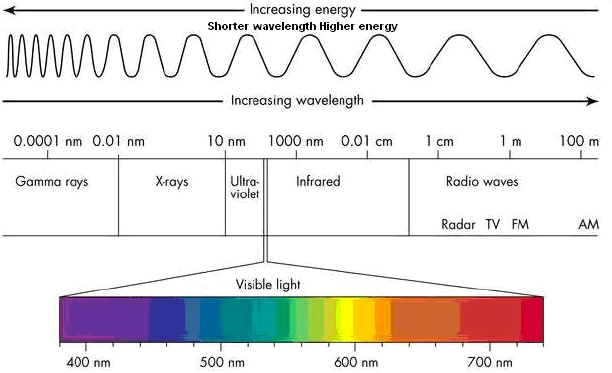Electromagnetic Spectrum

• It failed to explain the phenomenon of photoelectric effect, Compton effect and Raman effect.

Max Planck’s Quantum Theory

• Light emits from a source in the form of packets of energy called quanta or photon.
• The energy of a photon is E == hv, where h is Planck’s constant and v is the frequency of light.
• Quantum theory could explain photoelectric effect, Compton effect and Raman effect.
• Quantum theory failed to explain interference, diffraction and polarization of light.

De – Broglie’s Dual Theory

• Light waves have dual nature, wave nature according to Maxwell’s electromagnetic wave theory and particle nature according to Max-Planck’s quantum theory.
• Two natures of light are like the two faces of a coin. In anyone phenomena only its one nature appears.
• Energy of photon = hv = hc / λ
where, h = Planck’s constant 6.6 * 10<sup-34 J / s
de-Broglie wave equation is λ = h / p = h / mv
where h denotes Planck’s constant.

Superposition of Waves

When two similar waves propagate in a medium simultaneously, then at any point the resultant displacement is equal to the vector sum of displacement produced by individual waves.

y = y1 + y2

Interference of Light

• When two light waves of similar frequency having a zero or constant phase difference propagate in a medium simultaneously in the same direction, then due to their superposition maximum intensity is obtained at few points and minimum intensity at other few points.
• This phenomena of redistribution of energy due to superposition of waves is called interference of light waves.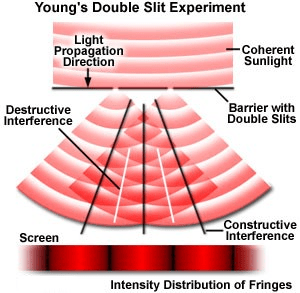• The interference taking place at points of maximum intensity is called constructive interference.
• The interference taking place at points of minimum intensity is destructive interference.

Fringe Width

• The distance between the centers of two consecutive bright or dark fringes is called the fringe width.
• The angular fringe width is given by θ = λ / d.
where λ is the wavelength of light d is the distance between two coherent sources.

Conditions for Constructive and Destructive Interference

➢ For Constructive Interference

• Phase difference, φ = 2nπ
• Path difference, Δx = nλ
where, n = 0, 1, 2, 3,…

➢ For Destructive Interference

• Phase difference, φ = (2n – 1)π
• Path difference, Δx = (2n – 1)π / 2
where, n = 1, 2, 3, …

If two waves of exactly same frequency and of amplitude a and b interfere, then amplitude of resultant wave is given by

R = √a2 + b2 + 2ab cos φ

where φ is the phase difference between two waves.

Rmax = (a + b)

Rmin = (a – b)

Intensity of wave

∴ I = a2 + b2 + 2ab cos φ

= I1 + I2 + 2 √I1 I2 cos φ

where I1 and I2 are intensities of two waves.

∴ I1 / I2 = a2 / b2 = ω1 / ω2

Where ω1 and ω2 are width of slits.

Energy remains conserved during interference.

Interference fringe width

β = Dλ / d

where, D = distance of screen from slits, λ = wavelength of light and d = distance between two slits.

Distance of nth bright fringe from central fringe xn = nDλ / d

Distance of nth dark fringe from central fringe x’n = (2n – 1) Dλ / 2d

Coherent Sources of Light

The sources of light emitting light of the same wavelength, the same frequency having a zero or constant phase difference are called coherent sources of light.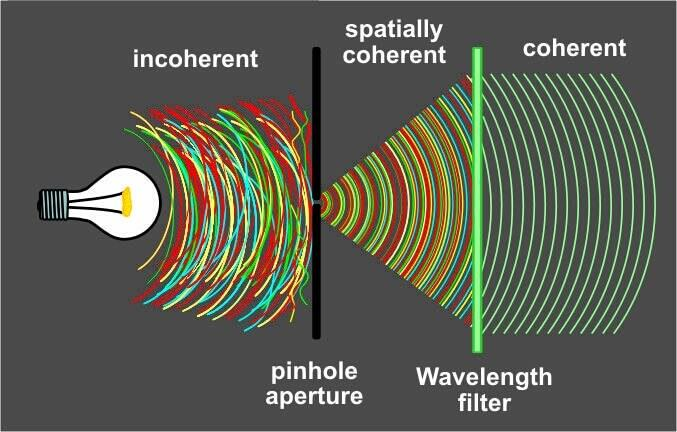Coherent sources of Light

When a transparent sheet of refractive index μ and of thickness t is introduced in one of the path of interfering waves, then fringe pattern shifts in that direction by a distance Y

Y = D / d (μ – 1) t = β / λ (μ – 1) t

where, β = fringe width.

➢ Fresnel’s Biprism

• It is a combination of two prisms of very small refracting angles placed base to base. It is used to obtain two coherent sources from a single light source.

➢ Llyod’s Mirror

• The shape of interference fringes are usually hyperbolic.
• When screen is held at 900 to the line joining focii of the hyperbola, the fringes are circular.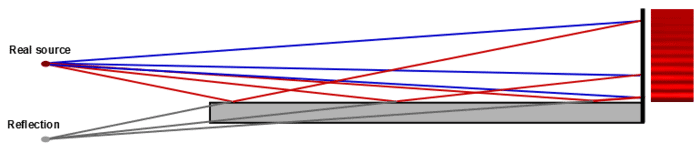Llyod's Mirror

• When distance of screen (D) is very large compare to the distance between the slits (d), the Cringes are straight.
Offer running on EduRev: Apply code STAYHOME200 to get INR 200 off on our premium plan EduRev Infinity!

## Physics For JEE

187 videos|516 docs|263 tests

,

,

,

,

,

,

,

,

,

,

,

,

,

,

,

,

,

,

,

,

,

;